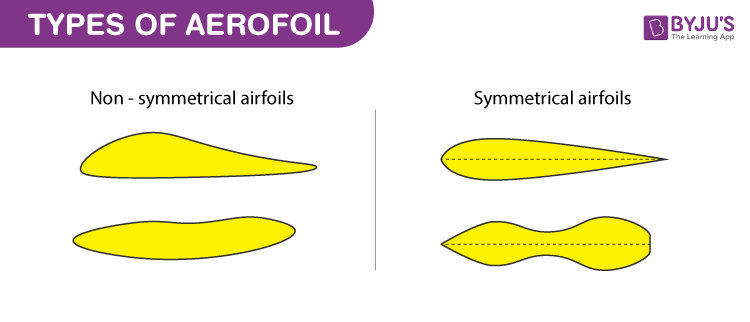# Aerofoil

## Definition of Aerofoil

Aerofoil or airfoil is defined as the cross-sectional shape that is designed with curved surface giving it the most favourable ratio between lift and drag in flight. Lift is the component such that the force is perpendicular to the direction of motion and drag is the component parallel to the direction of motion. A similar idea is used in the designing of hydrofoils which is used when water is used as the working fluid.

The designing of the aerofoil depends on the aerodynamic characteristics which further depends on the weight, speed and the purpose of the aircraft. These are dependent on certain terms that need to be defined to understand the design.

## Aerofoil Terminology

Following are the terms that are related to aerofoils:

• Chord: Chord is defined as the distance between the leading edge which is the point at the front of the aerofoil and has maximum curvature and the trailing edge which is the point at the rear of the aerofoil with maximum curvature along the chord line.
• Chord line: Chord line is defined as the straight line connecting the leading and trailing edges.
• Upper surface: Upper surface is also known as suction surface which is associated with high velocity and low static pressure.
• Lower surface: Lower surface is also known as pressure surface with higher static pressure.

Following are the terms used to describe the behaviour when the aerofoil is moving through a fluid:

• Aerodynamic center: The pitching moment is independent of lift coefficient and angle of attack (AOA) at this center.
• Center of pressure: The pitching moment is zero at this center.
• Angle of attack (AOA): The angle formed between a reference line on a body and the oncoming flow.
• Pitching moment: The moment or torque produced the aerodynamic force on the aerofoil.

### Lift Coefficient

Lift coefficient is a dimensionless coefficient giving relationship between the lift generated by a lifting body to fluid density, fluid velocity and the associated reference area. Mathematical representation is as follows:

$C_{L}\equiv \frac{L}{qS}=\frac{L}{\frac{1}{2}\rho u^{2}S}=\frac{2L}{\rho u^{2}S}$

Where,

CL: lift coefficient

L: lift force

S: relevant surface

q: fluid dynamic pressure

⍴: fluid density

u: flow speed

### Types of AerofoilFollowing are the types of aerofoils that are used:

• Symmetrical aerofoil: This has identical upper and lower surfaces such that the chord line and mean camber line are the same producing no life at zero AOA. These find applications in most of the light helicopters in their main rotor blades.
• Non-symmetrical aerofoil: It is also known as a cambered aerofoil. This has different upper and lower surfaces such that the chord line is placed above with large curvature. These have different chord line and chamber line. The advantages of non-symmetrical aerofoil is that the lift to drag ratio and stall characteristics are better and useful lift is produced at zero AOA. The disadvantages are that they are not economical and there is a production of undesirable torque.

To know more about other Physics related articles, stay tuned with BYJU’S.

Related Physics Articles: